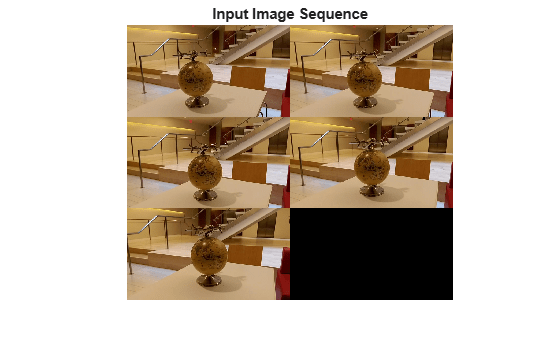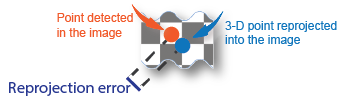# triangulateMultiview

3-D locations of world points matched across multiple images

## Syntax

``worldPoints = triangulateMultiview(pointTracks,cameraPoses,intrinsics)``
``````[worldPoints,reprojectionErrors] = triangulateMultiview(___)``````
``````[worldPoints,reprojectionErrors,validIndex] = triangulateMultiview(___)``````

## Description

example

````worldPoints = triangulateMultiview(pointTracks,cameraPoses,intrinsics)` returns the locations of 3-D world points that correspond to points matched across multiple images taken with calibrated cameras. `pointTracks` specifies an array of matched points. `cameraPoses` and `intrinsics` specify camera pose information and intrinsics, respectively. The function does not account for lens distortion.```
``````[worldPoints,reprojectionErrors] = triangulateMultiview(___)``` additionally returns the mean reprojection error for each 3-D world point using all input arguments in the prior syntax.```
``````[worldPoints,reprojectionErrors,validIndex] = triangulateMultiview(___)``` additionally returns the indices of valid and invalid world points. Valid points are located in front of the cameras.```

## Examples

collapse all

```imageDir = fullfile(toolboxdir("vision"),"visiondata","structureFromMotion"); imds = imageDatastore(imageDir);```

`data = load(fullfile(imageDir,"cameraParams.mat"));`

Get camera intrinsic parameters.

`intrinsics = data.cameraParams.Intrinsics;`

Compute features for the first image.

```I = im2gray(readimage(imds,1)); I = undistortImage(I,intrinsics); pointsPrev = detectSURFFeatures(I); [featuresPrev,pointsPrev] = extractFeatures(I,pointsPrev);```

```poseData = load("sfmCameraPoses.mat"); absPoses = poseData.absPoses;```

Create an `imageviewset` object.

```vSet = imageviewset; vSet = addView(vSet,1,absPoses(1),Points=pointsPrev);```

Compute features and matches for the rest of the images.

```for i = 2:numel(imds.Files) I = im2gray(readimage(imds,i)); I = undistortImage(I,intrinsics); points = detectSURFFeatures(I); [features,points] = extractFeatures(I,points); vSet = addView(vSet,i,absPoses(i),Points=points); pairsIdx = matchFeatures(featuresPrev,features,MatchThreshold=5); vSet = addConnection(vSet,i-1,i,Matches=pairsIdx); featuresPrev = features; end```

Find point tracks.

`tracks = findTracks(vSet);`

Get camera poses.

`cameraPoses = poses(vSet);`

Find 3-D world points.

```[xyzPoints,errors] = triangulateMultiview(tracks,cameraPoses,intrinsics); z = xyzPoints(:,3); idx = errors < 5 & z > 0 & z < 20; pcshow(xyzPoints(idx, :),AxesVisibility="on",VerticalAxis="y",VerticalAxisDir="down",MarkerSize=30); hold on plotCamera(cameraPoses, Size=0.2); hold off```## Input Arguments

collapse all

Matched points across multiple images, specified as an N-element array of `pointTrack` objects. Each element contains two or more points that match across multiple images.

Camera pose information, specified as a two-column table. You can obtain `cameraPoses` from an `imageviewset` object by using the `poses` object function.

ColumnDescription
`ViewID`View identifier in the `pointTrack` object, specified as an integer.
`AbsolutePose`Absolute pose of the view, specified as a `rigidtform3d` object.

Camera intrinsics, specified as a `cameraIntrinsics` object or an M-element vector of `cameraIntrinsics` objects. M is the number of camera poses. When all images are captured by the same camera, specify one `cameraIntrinsics` object. When images are captured by different cameras, specify a vector.

## Output Arguments

collapse all

3-D world points, returned as an N-by-3 matrix. Each row represents one 3-D world point and is of the form [x, y, z]. N is the number of 3-D world points.

Data Types: `single` | `double`

Reprojection errors, returned as an N-element vector. To calculate reprojection errors, first the function projects each world point back into each image. Then, in each image, the function calculates the distance between the detected and the reprojected point. Each element of the `reprojectionErrors` output is the average reprojection error for the corresponding world point in the `worldPoints` output.Validity of world points, returned as an M-by-1 logical vector. Valid points, denoted as a logical `1` (`true`), are located in front of the cameras. Invalid points, denoted as logical `0` (`false`), are located behind the cameras.

The validity of a world point with respect to the position of a camera is determined by projecting the world point onto the image using the camera matrix and homogeneous coordinates. The world point is valid if the resulting scale factor is positive.

## Tips

Before detecting the points, correct the images for lens distortion by using by using the `undistortImage` function. Alternatively, you can directly undistort the points by using the `undistortPoints` function.

 Hartley, Richard, and Andrew Zisserman. Multiple View Geometry in Computer Vision. 2nd ed. Cambridge, UK ; New York: Cambridge University Press, 2003.